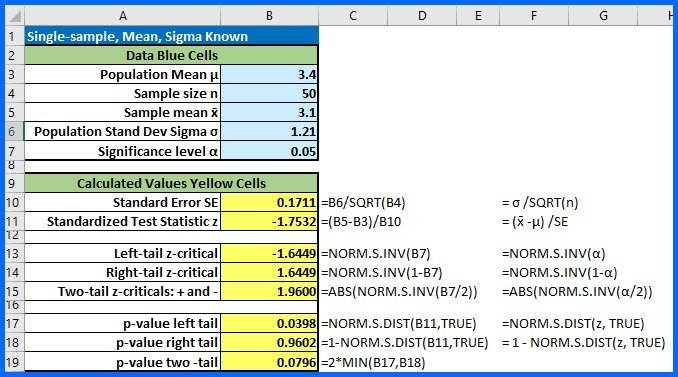Select Page

Here is a way to do a simple one-sample z test when we know the population standard deviation sigma. If this was a left tail test as indicated by an < in the alternative hypothesis, the standardized test statistic (z) of -1.753 falls in the rejection region below the z-critical of -1.645 and that would tell us to Reject the Null. The p-value for that same left-tail test is 0.0398 which is less than the significance level of 0.05, again telling us to reject the null.